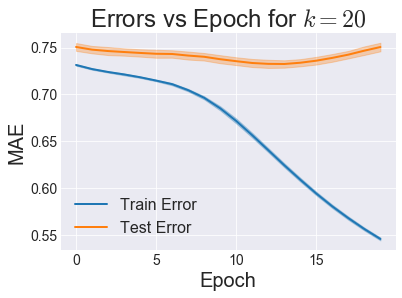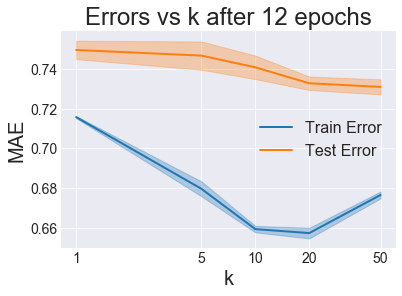This is the fifth post of a project on collaborative filtering based on the MovieLens 100K dataset. The remainder of this post is straight out of a Jupyter Notebook file you can download here. You can also see it here on GitHub.

Previously, I showed how to do matrix factorization using Alternating Least Squares (ALS). Now we'll attempt to factorize the matrix into the same mathematical form, but we'll use a different technique to get there.

Derivation details that give us the update equations we need can be found here. I'll just give the start and finish here.

We start with a loss function that looks like this:

$$L = \sum_{u,i}(r_{ui} - \hat{r}_{ui})^2 + \lambda_{b_u} \sum_u \lVert b_u \lVert^2 + \lambda_{b_i} \sum_i \lVert b_i \lVert^2 + \lambda_{x_u} \sum_u \lVert \mathbf{x}_u \lVert^2 + \lambda_{y_i} \sum_i \lVert \mathbf{y}_i \lVert^2$$

The first term is a sum of squared errors on the predicted rating, while all the other terms are regularizing penalties on too high of values, tunable by the 4 $\lambda$ parameters. $\hat{r}_{ui}$, the predicted rating for user $u$ on item $i$, is given by

$$\hat{r}_{ui} = \mu + b_u + b_i + \mathbf{x}_u^\top \cdot \mathbf{y}_i$$

With this setup, we can iterate over ratings, compute the gradient in the loss function for that point with respect to each parameter $b_u$, $b_i$, $\mathbf{x}_u$, and $\mathbf{y}_i$. As mentioned in the post linked above, the final update equations look like this

$$b_u^{t+1} = b_u^{t} + \eta (e_{ui} - \lambda_{b_u})b_u \\ b_i^{t+1} = b_i^{t} + \eta (e_{ui} - \lambda_{b_i})b_i \\ \mathbf{x}_u^{t+1} = \mathbf{x}_u^{t} + \eta (e_{ui} \mathbf{y}_i - \lambda_{x_u} \mathbf{x}_u) \\ \mathbf{y}_i^{t+1} = \mathbf{y}_i^{t} + \eta (e_{ui} \mathbf{x}_u - \lambda_{y_i} \mathbf{y}_i) \\$$

where $\eta$ is the learning rate (a parameter that controls the speed of descent down the gradients) and $e_{ui}$ is the prediction error given by $\hat{r}_{ui} - r_{ui}$.

The rest of this notebook goes through writing a class to fit and predict movie ratings using SGD, then using that class to tune model parameters.

In :
Expand Code

# 2. Load the Data¶

In :
Expand Code
First 5:

userId movieId rating timestamp
214 259 255 4 1997-09-19 23:05:10
83965 259 286 4 1997-09-19 23:05:27
43027 259 298 4 1997-09-19 23:05:54
21396 259 185 4 1997-09-19 23:06:21
82655 259 173 4 1997-09-19 23:07:23
Last 5:

userId movieId rating timestamp
46773 729 689 4 1998-04-22 19:10:38
73008 729 313 3 1998-04-22 19:10:38
46574 729 328 3 1998-04-22 19:10:38
64312 729 748 4 1998-04-22 19:10:38
79208 729 272 4 1998-04-22 19:10:38

In :
Expand Code

# 4. Determine how many epochs are necessary¶

In :
Expand Code
i_fold=0
i_fold=1
i_fold=2
i_fold=3
i_fold=4

In :
Expand CodeIt looks like around 12 is the optimal number of iterations to run before we start overfitting, so we'll use that from here on out.

# 5. Find Optimal $k$¶

In :
Expand Code
i_fold=0: k=1, k=5, k=10, k=20, k=50,
i_fold=1: k=1, k=5, k=10, k=20, k=50,
i_fold=2: k=1, k=5, k=10, k=20, k=50,
i_fold=3: k=1, k=5, k=10, k=20, k=50,
i_fold=4: k=1, k=5, k=10, k=20, k=50,

In :
Expand CodeThese are some very odd results that I'd want to look into given the time. I would expect training error to continually go down with increasing $k$, since that should allow for more overfitting to the training data. However, train error starts coming back up, even though test error is still going down! For now we'll just declare $k=50$ the winner since it has the lowest test error.